## Journey of Analytics

### Deep dive into data analysis tools, theory and projects

#### Category: Machine Learning (page 2 of 2)

Hello All,

In today’s tutorial we will apply 5 different machine learning algorithms to predict house sale prices using the Ames Housing Data.

This dataset is also available as an active Kaggle competition for the next month, so you can use this as a Kaggle starter script (in R). Use the output from the models to generate submission files for the Kaggle platform and view how well you fare on the public leaderboard.  This is also a perfect simulation for real-world analytics problem where the final results are validated by a customer / client/ third-party.

This tutorial is divided into 4 parts:

• Data Load & Cleanup
• Feature Selection
• Apply algorithms
• Using arithmetic / geometric means from multiple algorithms to increase accuracy

# Problem Statement:

Before we begin, let us understand the problem statement :

Predict Home SalePrice for the Test Data Set with the lowest possible error.

The Kaggle competition evaluates the leaderboard score based on the  Root-Mean-Squared-Error (RMSE)  between the logarithm of the predicted value and the logarithm of the observed sales price. So the best model will be one with a score of 0.

The evaluation considers log values so model is penalized for incorrectly predicting both expensive houses AND cheap houses. So the % of error deviation from real value matters, not just the \$ value of the homes.
E.g.: if the predicted value of homeprice was 42000\$ but actual value was 37000\$ , then \$-value error is only 5000\$ which doesn’t seem a lot. However, error % is ~13.52% .
On the contrary, imagine a home with real saleprice = 389411\$ , which we predicted to be 410000\$. \$ value difference is 20589\$, yet % error is only ~5.28% which is a better prediction.

In real-life too, you will frequently face situations where the sensitivity of predictions  is as important as value-accuracy.

As always,  you can download the code and data files from the Projects Page here , under the Feb 2017.

# Data Load & Cleanup:

In this step we perform the following tasks:

1. Load the test and training set.
2. Check training set for missing values.
3. Delete columns with more than 40% missing records. These include the variables Alley (1369 empty ), FireplaceQu (690), Fence (1179), PoolQC (1453), MiscFeature (1406) .
4. The other variables identified in step 1  are:
• LotFrontage , Alley , MasVnrType, BsmtQual , BsmtCond , BsmtExposure , BsmtFinType1, BsmtFinType2,  GarageType , GarageCond.
5. For columns with very few missing values, we choose one of 3 options:
• For categorical values, create a new level “unk” to indicate missing data.
• For columns (numeric or categorical) where the data tends to fall overwhelmingly in one category , mark missing values with this option.
• For numeric data, mark missing values with -1.
• NOTE, we will apply these rules on the test set also , for consistency, irrespective of whether there are missing values or not.
6. Repeat steps 2-5 for test set columns, since there may be empty cells for columns in test set which did not show up in the training set.  The variables we now identify include:
• MSZoning, Utilities, Exterior1st, Exterior2nd, MasVnrArea,
• BsmtFinSF1, BsmtFinSF2, BsmtUnfSF, TotalBsmtSF.
• BsmtFullBath. BsmtHalfBath, KitchenQual,
• GarageYrBlt, GarageQual, GarageFinish, GarageCars, GarageArea,
• SaleType.
7. We write the modified training and test sets to Excel so we can apply our models on the corrected data. ( This is especially helpful in real life if you need to fine tune your model over a few days time.

# Feature Selection:

The easiest way to check if a relationship exists is to use statistical functions: chisquare, anova or correlation.

Our target variable is “SalePrice” (numeric value) . So we will test all the other predictor variables against this factor to see if any relation exists and how much it affects the SalePrice.
For categorical predictors, we will use chisquare test, whereas for numeric predictors, we will use correlation.

Our dataset has 74 predictive factors (excluding SalePrice and Id), so we run a for loop to do a rough check. Relation exists only if p-values < 0.05.
If the predictor column is of type integer/numeric, we will apply correlation. If column = “character” , we apply chisquare.

We also add a column to identify variables of interest using the code below:

• If correlation value falls below -0.75 (high negative correlation) or above 0.75 (high positive correlation) then we mark the variable as “match”.
• If p-val from chisquare test falls below 0.05 then we mark it as “match”.

Using this “quack” approach, we quickly identify 19 variables of interest. These include Neighborhood, Building Type (single family, townhome, etc) , YearBuilt, Year Remodeled , Basement type (finished / unfinished), house area for first floor/ second floor / garage, number of bathrooms (both full / half) , no of cars garage can accommodate, sale type and sale condition.

NOTE, it is always a good idea to graphically visualize correlation functions as the above approach may miss out predictors with a non-linear relationship.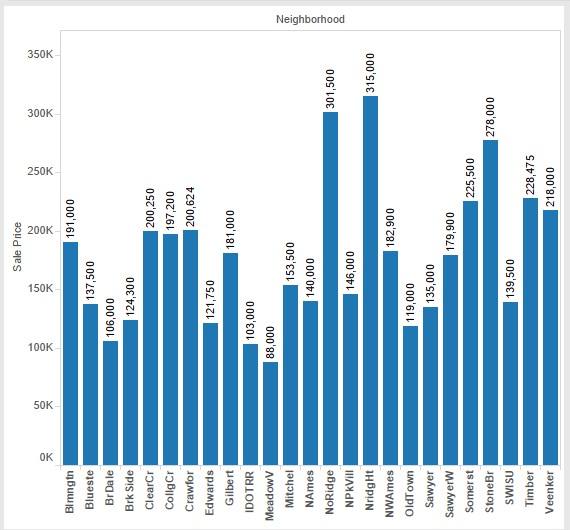Median House SalePrice by Neighborhood

# Apply Algorithms:

We apply the following machine learning algorithms:

1. Linear Regression Model.
2. Classification Tree Model.
3. Neural Network Model.
4. Random Forest algorithm model.
5. Generalized Linear Model (GLM)

We follow the same steps for all 5 models:
(Note, code and functions shown only for Linear Regression Model. Detailed functions and variables used for the other models are available in the R program files.)

1. Use the training set to create a formula.
2. Apply formula to predict values for validation set.
3. Check the deviation from true homeprices in terms of both median \$-value and % error.
4. Apply formula on test set and check rank/score on leaderboard.

The error rates for all 5 models are given in the table below: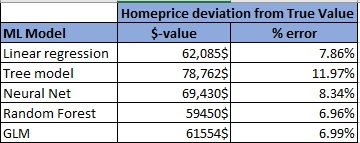home price table showing model error %

# Combining Algorithms to Improve Accuracy:

There are many scientific papers which show that combining answers from multiple models greatly improves accuracy. A simple but excellent explanation (with respect to Kaggle ) is given by MLwave.com founder and past Kaggle winner is  provided here. Basically, combining results from unrelated models can improve accuracy, even if individual models are NOT good at 100% accurate predictions.

Similar to the explanation provided in the link, we calculate the arithmetic mean to average the results from all 5 models .

# Summary:

We learnt how to clean and process a real-life dataset, select features of interest (and impact on target variable) apply 5 different machine learning algorithms.

The code for this tutorial is available here on the Projects page, under month of Feb.

Please take a look and feel free to comment with your own feedback or models.

In this post, we are going to learn how to apply a machine learning model for predictive analytics. We will create 5 models using different algorithms and test the results to compare which model gives the most accurate results. You can use this approach to compete on Kaggle or make predictions using your own datasets.

Dataset – For this experiment, we will use the birth_weight dataset from Delaware State Open Data Portal, which includes data from infants born in the period 2009-2016, including place of delivery (hospital/ birthing center/ home), gestation period (premature/ normal) and details about mother’s health conditions. You can download the data directly from the Open Data Link (https://data.delaware.gov/browse) or use the file provided with the code.

## Step 1 – Prepare the Workspace.

1. We clean up the memory of current R session, load some standard library packages (data.table, ggplot, sqldf, etc).
2. We load the dataset “Births.csv”.

## Step 2 – Data Exploration.

1. This step helps us understand the dataset – the range of values for variables, most common occurrences, etc. For our dataset, we look at a summary of birth years, birth weight and number of unique values.

2.  This is the point where we process for missing values and make a decision whether to ignore (entire column with large number of missing data), delete (very few records) or possibly replace it with median values. In this set however, there are no missing values that need to be processed.

3. Check how many unique values exist for each column.

## Step 3 – Test and Training Set

If you’ve ever competed on Kaggle, you will realize that the “training” set is the datafile used to create the machine learning model and the “test” set is the one where we use our model to predict the target variables.

In our case, we only have 1 file, so we will manually divide our set into 3 sets – one training set and one 2 test sets. (70% ,15%, 15% split) Why 2 test sets? Because it helps us better understand how the model reacts to new data. You can work with just one if you like. Just use one sequence command and stop with testdf command.

## Step 4 – Hypothesis Testing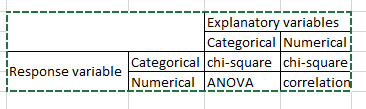statistical functions

In this step , we try to understand which predictors most affect our target variable using statistical functions such as ANOVA, chisquare, correlation, etc. The exact function you use can be determined using the table alongside.

Irrespective of which function we use, we assume the following hypothesis:
a) Ho (null hypothesis) – no relation exists. Ho is accepted if p-values if >= 0.05
b) Ha (alternate hypothesis) – relation exists. Ha is accepted if p-value < 0.05. If Ha is found true, then we conduct posthoc tests (for Anova and chisquare tests ONLY) to understand which sub-categories show significant differences in the relationship.

(1) Relation between birth_weight and mom’s_ethnicity exists since p-value < 0.05.

Using BONFERRONI adjustment and posthoc tests, we realize that mothers with “unknown” race are more likely to have babies with low birth weight, as compared to women of other races.

We also see this from the frequency table (below). Clearly only 70% of babies born to mothers of “unknown” race are of normal weight (2500 gms or above) compared to 92% babies from “other” race moms and 93% babies of White-race origins.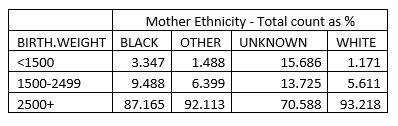mom ethnicity

(2) Relation between birth_weight and when prenatal_care started (first trimester, second, third or none) Although we see p-value < 0.05 Ha cannot be accepted because the posthoc tests do NOT show significant differences among prenatal care subsets.

(3) Relation between birth_weight and gestation period:

Posthoc tests show that babies in the groups POSTTERM 42+ WKS and TERM 37-41 WKS are similar and have higher birth weights than premature babies.
(4) We perform similar tests between birth_weight and multiple-babies (single, twins or triplets) and gender.

## Step 5 – Model Creation

We create 5 models:

• LDA (linear discriminant analysis) model with just 3 variables:
• LDA model with just 7 variables:
• Decision tree model:
• Model using Naïve Bayes theorem.
• Model using Neural Network theorem.

(1) Simple LDA model:

Model formula:

Make predictions with test1 file.

Examine how well the model performed.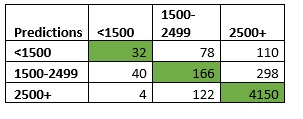lda_prediction-accuracy

From alongside table, we see that number of correct predictions (highlighted in green)

= (32+166+4150) / 5000

= 4348 / 50

= 0.8696

Thus, 86.96% predictions were correctly identified for test1! (Note, we will use the same process for checking all 5 models.)

Using a similar process, we get 88.4% correct predictions for test2.

#### (2) LDA model with just 7 variables:

Formula:

Make predictions for test1 and test2 files:

We get 87.6% correct predictions for test1 file and 88.57% correct for test2.

#### (3) Decision Tree Model

For the tree model, we first modify the birth weight variable to be treated as a “factor” rather than a string variable.

Model Formula:

Make predictions for test1 and test2 files:

We get 91.16% correct predictions for test1 file and 91.6% correct for test2. However, the sensitivity of this model is little low, since it has predicted that all babies will be of normal weight i.e “2500+” category. This is one of the disadvantages of tree models. If the target variable has a highly popular option which accounts for 80% or more records, then the model basically assigns everyone to it. (sort of brute force algorithm)

#### (4) Naive Bayes Theorem :

Model Formula:

Make predictions for test1 and test2 files:

Again we get model accuracy of 91.16% 91.6% respectively for test1 and test2 files. However, this model also suffers from a “brute-force” approach and has marked all babies with normal weight i.e “2500+” category. This reminds us that we must be careful about both accuracy and sensitivity of the model when applying an algorithm for forecasting purposes.

#### (5) Neural Net Algorithm Model :

Model Formula:

In the above formula, the “maxit” operation specifies a stop after maximum number of iterations, so that the program doesn’t go into an infinite loop trying to converge values. Since we have set the seed to 270, our formula converges after 330 iterations. With other “seed value” this number may be higher or lower.

Make predictions for test1 file:

Validation table (below) shows that total number of correct observations = 4592. Hence model forecast accuracy = 91.84%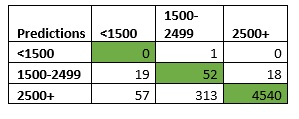nb_model_accuracy

Test with second file:

Thus, Neural Net models are accurate at 91.84% and 92.57% respectively for test1 and test2 respectively.

## Step 6 – Comparison of models

We take a quick look at how our models fared using a tabular comparison:  We conclude that neural network algorithm gives us the best accuracy and sensitivity.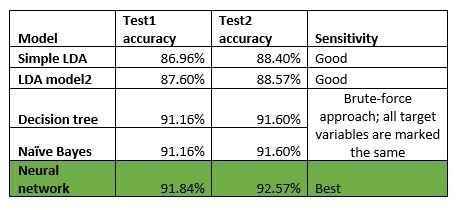compare data models

The code and datafiles for this tutorial are added to the New Projects page under “Jan” section. If you found this useful, please do share with your friends and colleagues. Feel free to share your thoughts and feedback in the comments section.

In this post we are going to analyze stock prices for company Facebook and create a linear regression model.

# Code Overview:

Our code performs the following functions. You can download the code here.

1. Load original dataset.
2. Add data to benchmark against S&P500 data.
3. Create derived variables. We create variables to store calculations for the following:
• Date values: split composite dates into day, month and year.
• Daily volatility : Price change between daily high and low prices or intraday change in price. A bigger difference signifies heavy volatility.
• Inter-day price volatility : Price change from previous day
4. Data visualization to validate relationship between target variable and predictors.
5. Create Linear Regression Model

# Step 1&2 – Load Datasets

We load stock prices for Facebook and S&P500. Note, the S&P500 index prices begin from 2004, whereas Facebook was listed as a public company only in May 2012.
We specify “all.x = TRUE” in the merge command to indicate that we do not want dates which are not present in the Facebook file.

fbknew = merge(fbkdf, sp5k, by = “Date”, all.x = TRUE)

Note, we obtained this data from the Yahoo! Finance homepage using the tab “Historical Data”.

# Step 3 – Derived Variables

a) Date Values:
The as.Date() function is an excellent choice to breakdown the date variable into day, month and year. The beauty of this function is that it allows you to specify the format of the date in the original data since different regions format it differently. (mmddyyyy / ddmmyy/ ..)

fbknew\$Date2 = as.Date(fbknew\$Date, “%Y-%m-%d”)
fbknew\$mthyr = paste(as.numeric(format(fbknew\$Date2, “%m”)), “-“,
as.numeric(format(fbknew\$Date2, “%Y”)), sep = “”)
fbknew\$mth = as.numeric(format(fbknew\$Date2, “%m”))
fbknew\$year = as.numeric(format(fbknew\$Date2, “%Y”))

b) Intraday price:

fbknew\$prc_change = fbknew\$High – fbknew\$Low

We have only calculated the difference between the High and Low, but since data is available for “Open” and “Close” you can calculate the maximum range as a self-exercise.
Another interesting exercise would be to calculate the average of both, or create a weighted variable to capture the impact of all 4 variables.

c) Inter-day price:
We first sort by date, using the order() function, and then use a “for loop” to calculate price difference from the previous day. Note, since we are working with dataframes we cannot use a simple subtraction command like x = var[i] – var[i-1]. Feel free to try, the error message is really beneficial in understanding how arrays and dataframes differ!

# Step 4 – Data Visualization

Before we create any statistical model, it is always good practice to visually explore the relationships between target variable (here “opening price”) and the predictor variables.

With linear regression model, it is more so, to identify if any variables show a non-linear (exponential, parabolic ) relationship. If you see such patterns you can still use linear regression, after you normalize the data using a log function.

Here are some of the charts from such an exploration between “Open” price (OP) and other variables. Note there may be multiple explanations for the relationship, here we are only looking at the patterns, not the reason behind it.

#### a) OP versus Trade Volume:

From chart below it looks like the volume is inversely related to price. There is also one outlier (last data point) . We use the lines() and lowess() functions to add a smooth trendline.
An interesting self-exercise would be to identify the date when this occurred and match it to a specific event in the company history (perhaps the stock split?)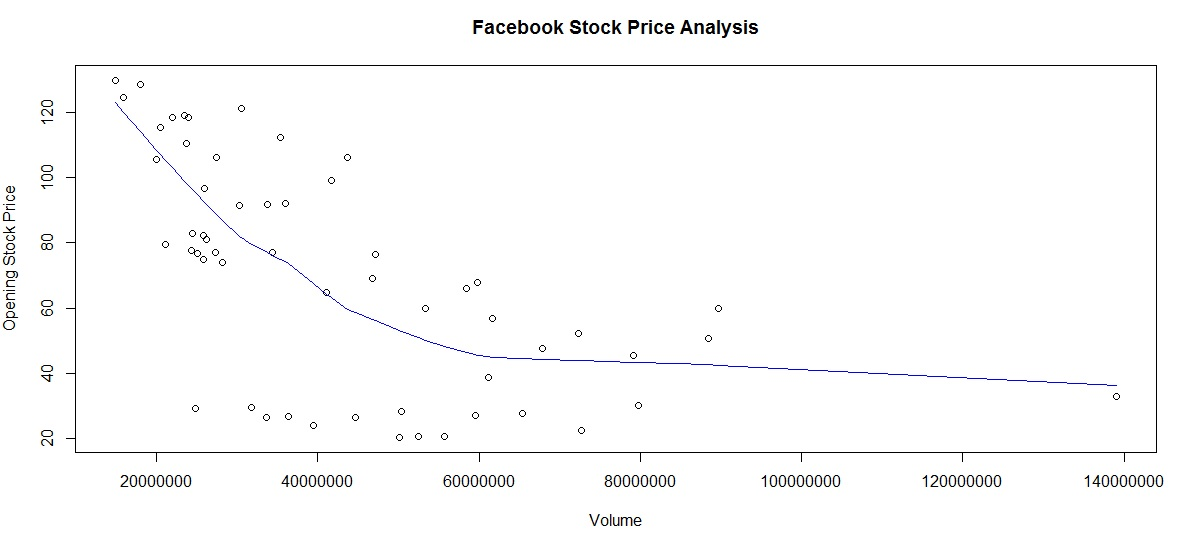Facebook Stock – Trading Volume versus Opening Stock Price

#### b) OP by Month & Year:

We see that the stock price has been steadily increasing year on year.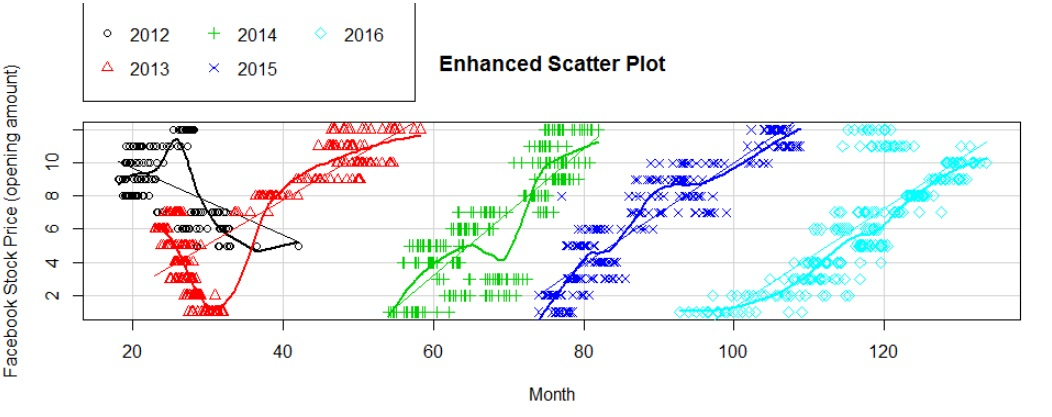Facebook price by month and year

#### c) OP versus S&P500 index price:

Clearly the Facecbook stock price has a linear relationship with S&P500 index price (logical too!)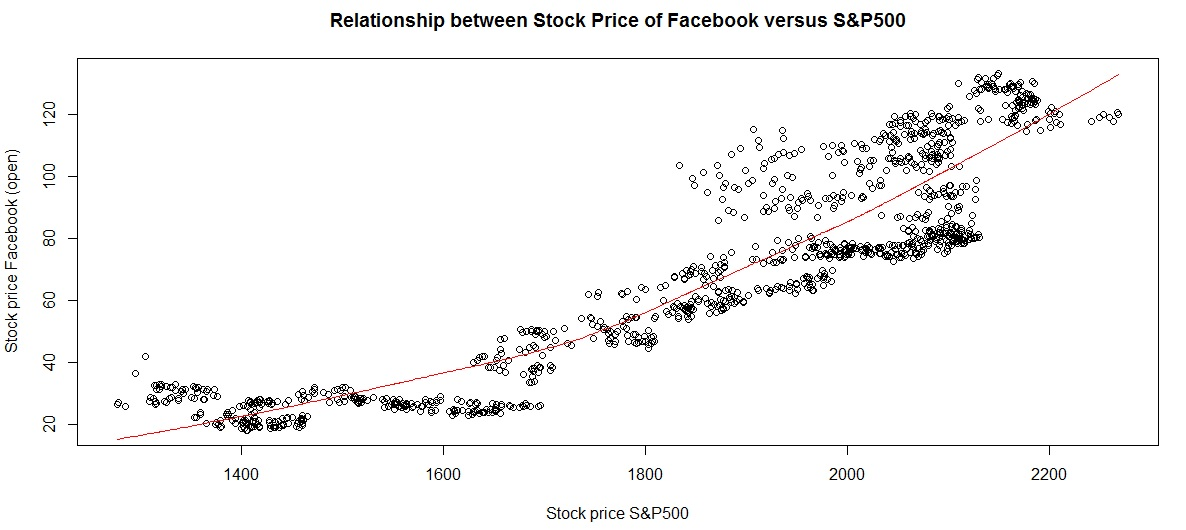Facebook stock price versus S&P500 performance

#### d) Daily volatility:

This is slightly scattered although the central clustering of data points indicates this as a fairly stable.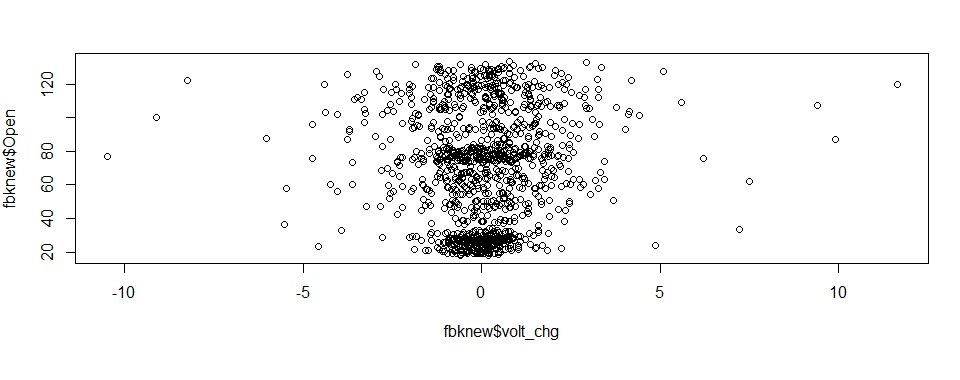Note, we use the sqldf package to aggregate data by monthyear / month for some of these charts.

# Step 5 – Linear Regression Model

Our model formula is as follows:

lmodelfb = lm(Open ~ High + Volume + SP500_price + prc_change +     mthyr + mth + year + volt_chg,     data = fbknew)
summary(lmodelfb)

We use the summary function to view the intercepts and identify the predictors with the biggest impact, as shown in table below: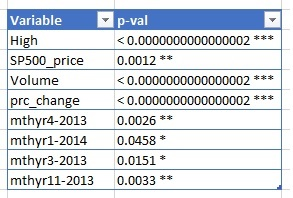We see that price is affected overall by S&P500, interday volatility and trade volume. Some months in 2013 also showed a significant impact.
This was our linear regression data model. Again, feel free to download the code and experiment for yourself.

Feel free to add your own observations in the comments section.

In the last few posts, we saw standalone analytics projects to perform sentiment analysis, visually explore large datasets for insights  and create interesting Shiny applications.

In the coming months however, we will cover how to implement machine learning algorithms in depth. We will explore the underlying concepts behind the algorithm, (why and how the formula works) , implement using a real-world Kaggle dataset and also learn about limitations and advantages.

What algorithms will we cover?

There are many algorithms to choose from, and this infographic from ThinkBigData provides an excellent and comprehensive list. Feel free to use as a handout or print one for your cubicles! For our purposes, we will cover two algorithms from each category.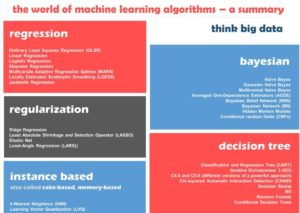Categories of machine learning algorithms. Source – ThinkBigData.com, by author Anubhav Srivastava.

Quick FAQ – selecting algorithm in practice

Many readers often ask, “how do I understand which algorithm to select? ” And this is also where new programmers often get stuck.

The long-winded answer is there is no secret sauce, and unfortunately often comes from experience or the problem definition itself.

The above answer is not very satisfying, so here are two “cheat-sheet” answers:

1. A good approximation is given by this infographic by Microsoft Azure is a great example.  Download it from Link here.
2. Regression is a very common and flexible model, so the table below provides idea to create a base model based on whether your target variable is qualitative (numeric) or categorical (e.g gender or country)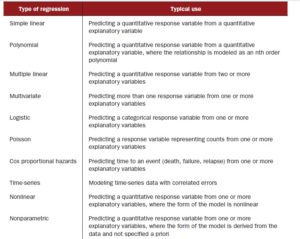regression algorithms based on target variables